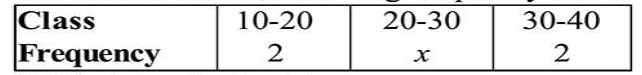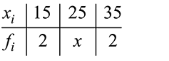# If a variance of the following frequency distribution :Question:

If a variance of the following frequency distribution :is 50, then $x$ is equal to_____.

Solution:$\bar{x}=\frac{\Sigma f_{i} x_{i}}{\Sigma f_{i}}=\frac{30+70+25 x}{4+x}=25$

$\sigma^{2}=\frac{\Sigma f_{i} x_{i}^{2}}{\Sigma f_{i}}-(\bar{x})^{2}$

$\Rightarrow 50=\frac{450+625 x+2450}{4+x}-625$

$\Rightarrow 675=\frac{2900+625 x}{4+x} \Rightarrow 50 x=200$

$\therefore x=4$